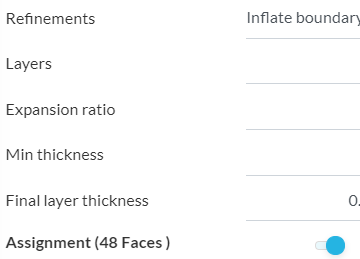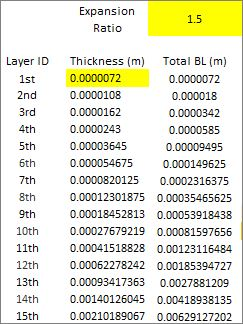# Mesh study external aerodynamics problem

#21

I have some questions about the layer refinement. In this mesh you did, what value of Y+ were you aiming? And what value of expansion rate you used?

#22

@math_marques96 it depends on what turbulence model you plan on using. I would start with the \kappa \text - \epsilon model and target a y^+ of about 50 with a growth factor of around 1.2 - 1.3 and a last layer thickness somewhere around 83\% of your standard surface mesh size. After running a few cases with \kappa \text - \epsilon you will know whether or not separation is an issue. If so, I would switch to the \kappa \text - \omega\,SST model and then target 1 \leq y^+ \leq 5 in the areas where the separation occurs.

The first model will get you about 90\% of the way there and the second will get you that last 10\%. It all depends on your time requirements and what you are looking to get out of the simulation.

#23

Hello,
I made some changes in the layers refinement and acquired a better mesh. I am in doubt about a layer parameter.
I found this forum Boundary layer inflation thickness and it was not clear for me if what parameter is the “final layer thickness”. On this forum there is also a “first layer thickness” parameter that not appear in my simulation.
I uploaded this draw of a boundary layer, to show my question, the parameter “final layer thickness” is the A or B? Is it possible to choose between using the “final layer thickness” or “first layer thickness”, I did not understand if it is the same also.

#24

Hey @math_marques96!

• firstLayerThickness - first layer in the boundary layer (next to the wall) \rightarrow B
• finalLayerThickness - last layer in the boundary layer \rightarrow A

Hope that helpsJousef

#25

Thank you very much!!

In my simulation, the “final layer thickness” parameter appears. Is it possible to I select the “first layer thickness” parameter instead? I am not finding where I can select this option.

#26

Our PowerUser @anirudh2821998 has posted a good procedure for this here: Boundary layer inflation thickness - if anything is missing for your calculations, please let us know and we will fill this gap!

Best,

Jousef

#27I read the post and did not find where can I change the “final layer thickness” to the"first layer thickness". This is what shows in my simulation:

#28

That is impossible to do, you must create a table like this (and you have to read and understand how the mesh parameter ‘Layer Size’ affects the value of what you enter in the ‘Final Layer Thickness’ (FLT) parameter):If ‘Layer Size’ is ON (also known as Relative Layering), then FLT is a dimensionless Ratio of the final prism cell size to the adjoining volume mesh cell.

If you choose to use Relative Layering (I do) then you need a further table that lets you pick your FLT ratio based on the surface level refinement, which you choose to refine all the faces that you are putting a boundary layer on (I generally use an FLT ratio of ~0.2 to ~0.4 but that is not a hard rule):

If ‘Layer Size’ is OFF, then FLT is an absolute thickness in meters of the final layer that touches the volume mesh cell.

#29

Here are the steps to make the desired number of inflation layers

1. Use any y+ calculator to get the value of first layer(nearest to wall). Just feed in some values asked by the calculator and you will get the suitable thickness of first layer. I prefer to use this calculator- http://www.pointwise.com/yplus/

2. Decide a suitable expansion ratio and number of inflation layers. Then determine the thickness of farthest layer —a*(r^(n-1))
a= first layer thickness, r=expansion ratio and n= number of layers

3. Check whether relative sizing option is on/off. I usually prefer my relative sizing option to be off.

Thanks
Ani